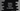# How to convert hours to seconds in Python## How to convert hours to seconds in Python:

In this post, we will learn how to convert hours to seconds in Python. The program will take the hours value as an input from the user, convert it to seconds and print it out.

This is a simple python example program and you will learn how to do simple mathematical calulations in python, how to read user inputs in python and how to print values on console in python.

### Algorithm for the program:

To solve this problem, we need use a simple mathematical formula, i.e. we need to multiply the value of hour by 60. That’s it. For multiplication, we can use the * operator.

### Python program:

Below is the complete python program:

``````hour = int(input('Enter the hour value: '))
seconds = hour * 60

print('seconds= {}'.format(seconds))``````

Here,

• We are taking the value of hour as input from the user and storing that value in the variable hour.
• This value is converted to seconds by multiplying it with 60. It is stored in the variable seconds.
• The last line is printing the value of the seconds variable.

### Sample output:

If you run this program, it will print output as like below:

``````Enter the hour value: 3
seconds= 180``````

### By using a different method:

We can also use a different method to do the exact same thing. The advantage of using a different method is that it will be easier to call that method from different places, e.g. we can place that method in an utility file and call that method from different places in the program.

``````def hour_to_seconds(hour):
return 60 * hour

hour = int(input('Enter the hour value: '))

print('seconds= {}'.format(hour_to_seconds(hour)))``````

Here, we are using a different method called hourtoseconds to convert the hour value to seconds. We can put this method in a different file and call it from anywhere we want.

If you run this program, it will print similar output as like the above program.

### Conclusion:

In this post, we learned how to convert a hour to seconds in python with examples. Using a different method for utility tasks is preferred than repeating the same code in multiple places.